# Adding Scalar Multiples of Vectors in a Graph

Paymemoney

## Homework Statement

Draw the vector $$\vec{C} = \vec{A}+2\vec{B}$$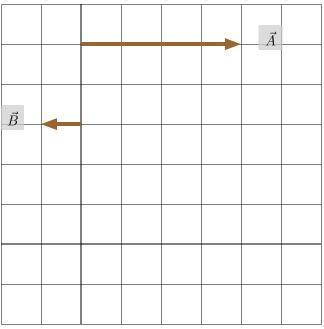None

## The Attempt at a Solution

This is what i have i don't know if it is correct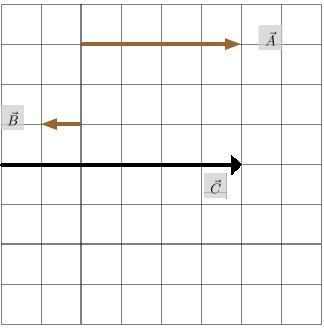P.S

## Answers and Replies

Homework Helper
Hi Paymemoney!No, that's not right.

When adding vectors, go to the end of the first vector, then tack on the second vector.Paymemoney
ok so is this what it should look like: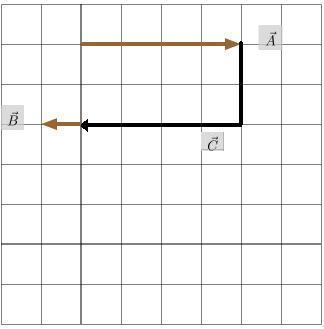Homework Helper
Why have you bent it??

Put it back and start again!

(if it's broken, you'll have to pay for it)

Paymemoney
how i do this without bending it? So is it only meant to go horizontally?

Homework Helper
how i do this without bending it? So is it only meant to go horizontally?

Yes, of course. And to the left.

Paymemoney
so would this be correct.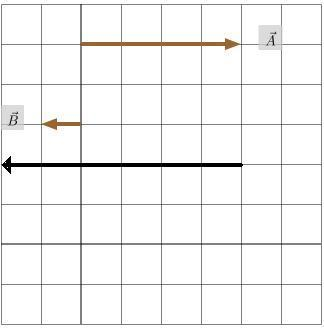does it matter where you place the vector, because when i placed it in the middle it was incorrect??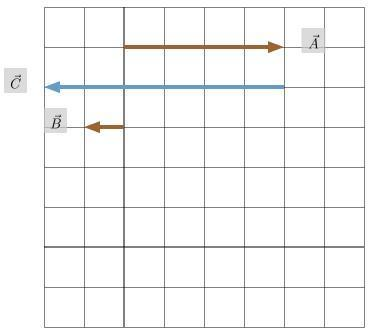Homework Helper
Hi Paymemoney!(just got up :zzz: …)
so would this be correct.

Nooo …

I think I need to give you the answer:

To add two vectors, you must place the "tail" of the second vector against the "head" of the first vector: the sum is then the vector to that point (from where you started).​

(or the other way round, of course: place the "tail" of the first vector against the "head" of the second vector: it makes no difference)

So in this case, you draw A which is an arrow going 4 to the right, then you draw B which is an arrow going 2 to the left, and you draw that 2 starting at the end of the 4, so you end up with an everall vector of A + 2B being 2 to the right.

(this is just like adding vectors to make a triangle, except that in this case the triangle is flat)

Paymemoney
So in this case, you draw A which is an arrow going 4 to the right, then you draw B which is an arrow going 2 to the left, and you draw that 2 starting at the end of the 4, so you end up with an everall vector of A + 2B being 2 to the right.
ok, this is what i got from your explanation, so just to make sure i have interpreted your explanation correctly here it is again(hopefully correct -_-).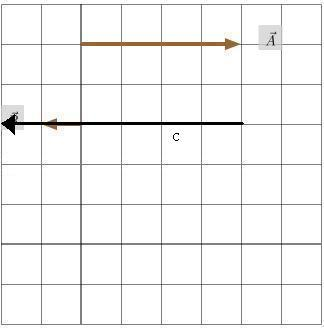(this is just like adding vectors to make a triangle, except that in this case the triangle is flat)

yeh, I'm used to looking at the full triangle.

Homework Helper
ok, this is what i got from your explanation, so just to make sure i have interpreted your explanation correctly here it is again(hopefully correct -_-).

I don't understand what you've done here.Start on the axis, draw a blue line for A, then from the end of that draw a red line for 2B. Now draw a green line from the axis to the end of the red line (as if you were completing a triangle).

Paymemoney
ok here it is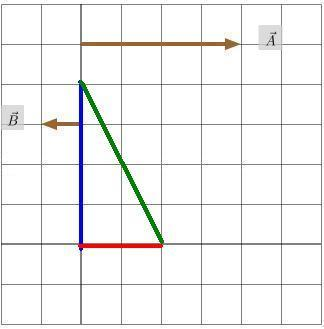why don't you draw it for me coz i don't think i'm getting anyway.

Paymemoney
after thinking more about it, i have come to a conclusion that this may be the answer but i'm not sure: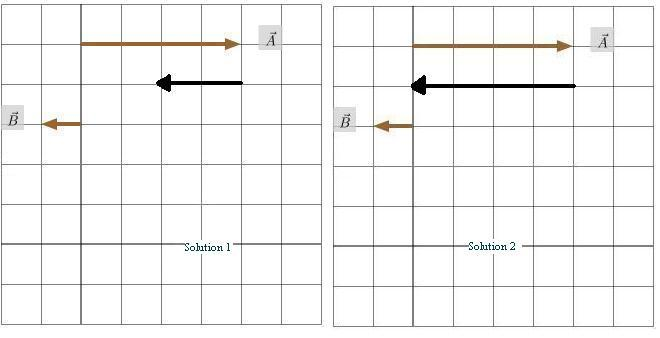Mentor
No, not right. A is 4 units long and points to the right. B is 1 unit long and points to the left. 2B is 2 units long and points to the left.

For the sum A + 2B, start at 0, go 4 units to the right. Now tack 2B on. Where do you end up? The vector from 0 to where you ended up is A + 2B.

Since all vectors involved here are horizontal, the sum will be a horizontal vector: one that points either to the right or to the left.

Paymemoney
What do you mean by "Now tack 2B on"?

Homework Helper
2B or not 2B ?

What do you mean by "Now tack 2B on"?

Mark44means "tail to head" (as I also explained in post #8)

Paymemoney
ok, i had another go at it, and this is what i got:Last edited:
Mentor
We're 16 posts into this thread, and you still aren't getting it. Why do you have two diagrams? Are we supposed to pick one?

In the right diagram, the resultant (black) vector starts at the right place, but it's too short. In the left diagram, the resultant vector is the right length, but it starts at the wrong place.

Paymemoney
well i finally got the answer =___=, yeh it took me awhile because i didn't read the answer you gave me correctly. thanks for the help anyways.